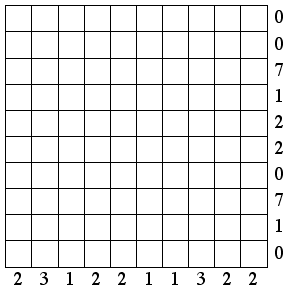2002 U.S. Championship Puzzles

1. Equations

Place the digits 1 through 9 (each used exactly once) into the squares so that the numbers that fit inside the circles to make the equations true are the same.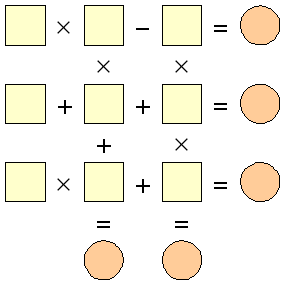2. Balancing Act

Assign the values 1 to 10 to the weights in the diagram so that everything balances as shown. Each number will be used exactly once.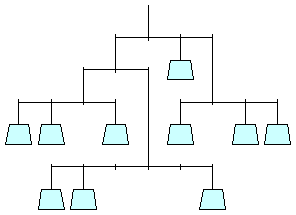3.-4. Triangle Math

Put the digits 1 through 9, each used once, into the circles so that the three equations reading left to right are true.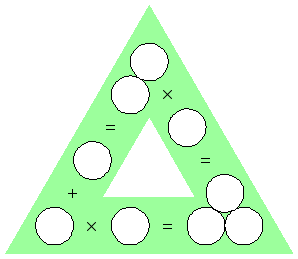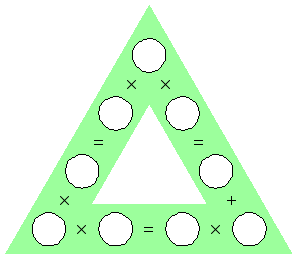5. Path Battleships

Locate the 10 ships so that the remaining white space can be completely filled by a single, closed loop, connecting squares horizontally or vertically.